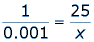Math Central - mathcentral.uregina.caMath Beyond Schoolreturn to topRatios and Proportions Used in Medicine Nurses also use ratios and proportions when administering medication.  Nurses need to know how much medicine a patient needs depending on their weight.   Nurses need to be able to understand the doctor’s orders.  Such an order may be given as: 25 mcg/kg/min.  If the patient weighs 52kg, how many milligrams should the patient receive in one hour?  In order to do this, nurses must convert micrograms (mcg) to milligrams (mg).  If 1mcg = 0.001mg, we can find the amount (in mg) of 25mcg by setting up a proportion.By cross-multiplying and dividing, we see that 25mcg = 0.025mg.  If the patient weighs 52kg, then the patient receives 0.025(52) = 1.3mg per minute.  There are 60 minutes in an hour, so in one hour the patient should receive 1.3(60) = 78mg.  Nurses use ratios and proportions daily, as well as converting important units.  They have special “shortcuts” they use to do this math accurately and efficiently in a short amount of time.
 Math Central is supported by the University of Regina and The Pacific Institute for the Mathematical Sciences.about math central :: site map :: links :: notre site français